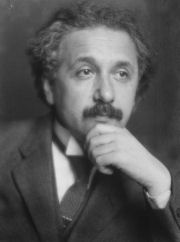# Einstein Field Equations (General Relativity)$\LARGE G_{\mu\nu}+g_{\mu\nu}\Lambda=\frac{8{\pi}G}{c^4}T_{\mu\nu}$

## The Terms$G_{\mu\nu}=R_{\mu\nu}-\frac{1}{2}Rg_{\mu\nu}$ - Einstein tensor$R_{\mu\nu}$ - Ricci curvature tensor$R$ - Scalar curvature$g_{\mu\nu}$ - Metric tensor$\Lambda$ - Cosmological constant$G$ - Newton's gravitational constant$c$ - Speed of light$T_{\mu\nu}$ - Stress-energy tensor

## What Does It Mean?

The Einstein Field Equations are ten equations, contained in the tensor equation shown above, which describe gravity as a result of spacetime being curved by mass and energy.$G__{\mu\nu}$ is determined by the curvature of space and time at a particular point in space and time, and is equated with the energy and momentum at that point. The solutions to these equations are the components of the metric tensor$g_{\mu\nu}$, which specifies the spacetime geometry. The inertial trajectories of particles can then be found using the geodesic equation.

### Did You Know?

The equations must be wrong! Although the theory and the equations have passed every test, they are intrinsically incompatible with quantum theory (which has also passed every experimental test). The problem is that the equations require the energy and momentum to be defined precisely at every space time point, which contradicts the uncertainty principle for quantum states. This is not a just a problem at high energies or short distances, it is a conceptual incompatibility that applies in every lab.

### Further information at Warwick

General Relativity is introduced in the third year module "PX389 Cosmology" and is covered extensively in the fourth year module "PX436 General Relativity".